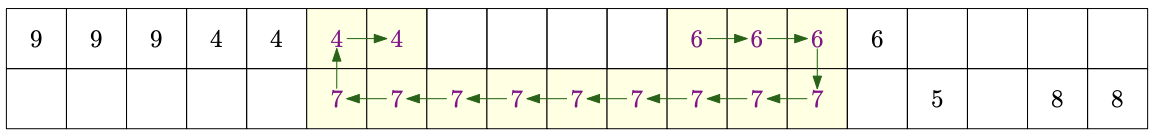시간 제한메모리 제한제출정답맞힌 사람정답 비율
5 초 (추가 시간 없음) 1024 MB0000.000%

## 문제

A marathon race is being planned in the beautiful countryside. The course will be somewhere along a long, bidirectional, road. The organizers want to determine exactly where along this road the race should be in order to maximize the experience for the runners, so that they get to enjoy as much beautiful scenery as possible and are distracted from their tired limbs. The scenery varies in beauty depending on where on the road you are, and also in which direction you are running. Because of this, the organizers are fine with having the runners make up to two U-turns, as long as no part of the road is used more than once in each direction.

We model the road of length $m$ meters as a rectangular grid of size $2 \times m$, where each cell has a non-negative "beauty" value associated with it. The columns represent each meter of the road ordered from start to to end. The top row in a column represents the beauty for this part of the road when running in the direction towards the end of the road, and the bottom row in a column represents the beauty when running towards the start of the road. A race of length $x$ is then some set of exactly $x$ of the cells in the grid.   Those $x$ cells must form a path in the grid where no cell is visited more than once, we only move to the right or down from cells in the top row, and we only move to the left or up from cells in the bottom row.  See Figure M.1 for an example race. The "total beauty" of a race is the sum of the beauty values of the included cells.

The road is long, so rather than providing a list of all of the $2m$ beauty values, each side of the road is divided into a small number of segments, where the cells within a segment have some constant beauty value (and cells with beauty $0$ are simply omitted).Figure M.1: Illustration of Sample Input 1. The numbers in the cells indicate the beauty value for each meter of the road (with omitted values being $0$). The highlighted cells and arrows mark the optimal race, involving two U-turns.

## 입력

The first line of input contains the three integers $m$, $x$ and $n$ ($1 \leq m \leq 10^9$, $1 \leq x \leq 2 m$, $0 \leq n \leq 200$), the length of the road, the length of the race and the number of segments.

This is followed by $n$ lines describing the segments. Each such line contains three integer $a, b, v$ ($0 \leq a, b \leq m$, $1 \leq v \leq 10^9$, and $a \ne b$), describing a segment with endpoints $a$ and $b$ having beauty value $v$.  If $a < b$, this is the segments of cells in the top row of the grid in the range $[a, b)$, and if $a > b$, this is the segments of cells in the bottom row of the grid in the range $[b, a)$.

The parts of the road that are not covered by any segments have beauty value $0$.  Each cell in the grid is covered at most once (that is, there are no overlapping segments in the same direction).

## 출력

Output the maximum possible total beauty the race can have.

## 예제 입력 1

19 14 6
14 5 7
11 15 6
3 7 4
16 15 5
19 17 8
0 3 9


## 예제 출력 1

89


## 예제 입력 2

100000 42195 2
30000 60000 500000000
40000 10000 1000000000


## 예제 출력 2

35548500000000


## 출처• 문제를 만든 사람: Jimmy Mårdell